College Physics : Faraday's Law of Induction

Example Questions

Example Question #2 : Electromagnetics

A single string of wire has a resistance of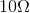. If the wire is connected to a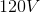power source, what is the strength of the magnetic field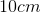away from the wire?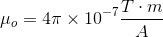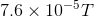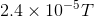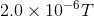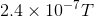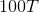Explanation:

So this is all about the magnetic field strength around a current carrying wire.

The equation for this is: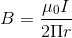But you must use Ohm's Law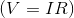in order to find the current in the wire.

Since the wire hasof resistance and the voltage through the wire is, that means the current in the wire is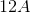.

Being sure to changeinto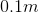, plug everything in and get the answer, which isAll College Physics Resources